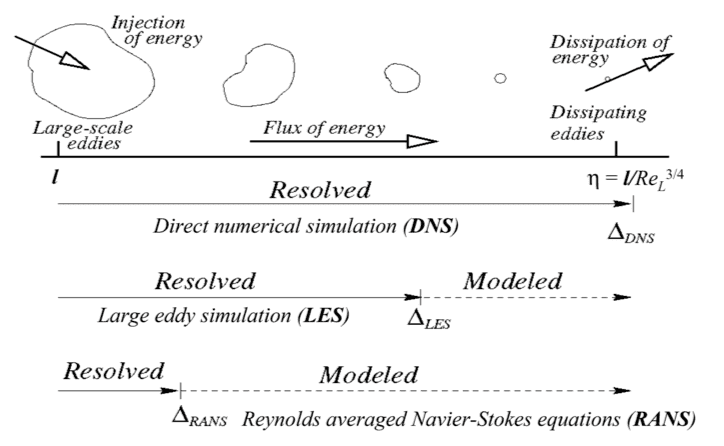# Chaos & Scales in Turbulent Flows

Suppose you want to solve the Navier-Stokes equation for an incompressible turbulent flow. This is, in principle, possible. However, for higher Reynolds number, this isn't really feasible since the smallest eddy sizes are really small.

First question
1) Why would this prevent you from computing a solution? I can't actually imagine in my head the computation procedure, and why having tiny eddies is different from just having small eddies? Surely you have to compute the whole flow field anyway?

Second & Third questions
Turbulent flows have two important characteristics:
> acute sensitivity to initial conditions, meaning a precise deterministic prediction of the flow is impossible.
> velocity has a reproducible (and hence, predictable?) mean component, and a chaotic, fluctuating component.

2) Doesn't the first statement mean that DNS is useless anyway? If you can't specify initial (and boundary and material) conditions for the mathematical model (the Navier-Stokes equations) to be exactly the same as the real life, then your calculated solution will be wrong?

3) Is the fact that the mean is reproducible (predictable) the basis of the RANS models? Like, we can predict the mean component, and we make some assumptions (like eddy-viscosity hypothesis) about the fluctuating?

I would have written three separate questions, but they are all related – how to predict the velocity field in a turbulent flow field?

Thanks for the post! This is an automated courtesy bump. Sorry you aren't generating responses at the moment. Do you have any further information, come to any new conclusions or is it possible to reword the post?

Gold Member
1) Why would this prevent you from computing a solution? I can't actually imagine in my head the computation procedure, and why having tiny eddies is different from just having small eddies? Surely you have to compute the whole flow field anyway?

In a turbulent flow, there are many scales, as you have pointed out, and generally, the difference in scales between the largest and the smallest scales is many orders of magnitude. With a DNS, if your grid does not resolve down to the smallest scales of physical importance (which typically range from nanometers to micrometers), then you are effectively averaging out or ignoring part of the physics. If you don't resolve the largest scales (often measured in meters), then you do the same thing, only the situation is worse because those contain most of the energy. Furthermore, as ##Re## increases, the separation in scales becomes greater and the time separation required in stepping the solution decreases. So the problem essentially boils down to the number of grid points and time points that you need in order to resolve all of the relevant scales. As it turns out, the number of operations required to solve such a problem on a computer scales as ##Re^3##, so it rapidly approaches a point where even modern supercomputers take an impractical length of time to solve a problem.

2) Doesn't the first statement mean that DNS is useless anyway? If you can't specify initial (and boundary and material) conditions for the mathematical model (the Navier-Stokes equations) to be exactly the same as the real life, then your calculated solution will be wrong?

Not necessarily. In order to make use of the data from a turbulent flow for the overwhelming majority of applications, the important quantities are the mean fields. Even if the initial conditions from two simulations are slightly different due to computing limitations, they will still converge around the same mean, so the DNS is still useful. Now, knowing how the fluctuating quantities behave statistically is important in determining how those mean fields differ from the laminar case when it comes to turbulence models, but knowing exactly what those fluctuating values are an one instant of time is not generally important.

You are right to be thinking about initial conditions, though. Any real flow typically does not start out turbulent, and small changes to initial conditions can have big implications on where and how the flow ultimately transitions to turbulence, and in that case, sometimes the difference in the initial conditions leading into the turbulent regime can meaningfully affect the characteristics of the turbulence downstream, at least initially. For a given ##Re##, however, that turbulent flow should eventually reach a fully-developed state that is statistically independent of the initial conditions. By then, from an applications standpoint, the rest of the flow is likely already so different that the initial conditions were still very important. For example, the difference in initial conditions will dramatically alter the drag on an aircraft, even if the turbulent boundary layer always eventually reaches the same state statistically speaking.

3) Is the fact that the mean is reproducible (predictable) the basis of the RANS models? Like, we can predict the mean component, and we make some assumptions (like eddy-viscosity hypothesis) about the fluctuating?

Yes, the fact that the mean is reproducible is the basis for turbulence models, in a sense. However, turbulence models are essentially designed to compute the mean flow while modeling the fluctuating components in order to suitably modify the mean flow, so you have it backward (at least in terms of how you have written it here). Basically, as long as we know something about the fluctuations, we can use that in a turbulence model to approximate the mean flow, and from that extract more meaningful quantities like shear stress.

•humphreybogart
Thank you for your explanation. I found the following graphic which also helps.